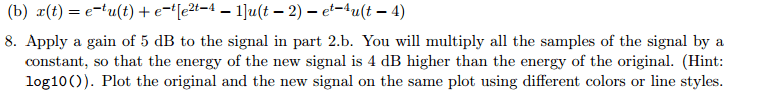# Applying a gain of dB to a signal (function) help?

## Homework Statement## Homework Equations

The ratio of the parameters $\frac{E_1}{E_2}$ to find the gain (in dB), 10$\times$log10$\frac{E_1}{E_2}$.

"To determine the gain factor in part 8, first think about what happens to the energy when you multiply the signal by a constant factor. Then use the logarithmic formula above to solve for the gain factor."

## The Attempt at a Solution

Since energy is square of integral of a f(x), I am thinking if f(x) is multiplied by constant factor then energy will be square of that factor greater. I already have E1 of the problem, which is the energy of f(x) above = 2.99. I am confused I think because problem says 5dB then 4dB. anyway..if I assume gain of energy is equal to 4dB i have:

4dB = 10$\times$log10$\frac{E_1}{E_2}$
0.4 = log10$\frac{E_1}{E_2}$
$\frac{E_1}{E_2}$ = 2.512 → E2 = $\frac{2.99}{2.512}$ = 1.19

but why is the energy less than the original though? am i doing this right so far??

berkeman
Mentor

## Homework Statement## Homework Equations

The ratio of the parameters $\frac{E_1}{E_2}$ to find the gain (in dB), 10$\times$log10$\frac{E_1}{E_2}$.

"To determine the gain factor in part 8, first think about what happens to the energy when you multiply the signal by a constant factor. Then use the logarithmic formula above to solve for the gain factor."

## The Attempt at a Solution

Since energy is square of integral of a f(x), I am thinking if f(x) is multiplied by constant factor then energy will be square of that factor greater. I already have E1 of the problem, which is the energy of f(x) above = 2.99. I am confused I think because problem says 5dB then 4dB. anyway..if I assume gain of energy is equal to 4dB i have:

4dB = 10$\times$log10$\frac{E_1}{E_2}$
0.4 = log10$\frac{E_1}{E_2}$
$\frac{E_1}{E_2}$ = 2.512 → E2 = $\frac{2.99}{2.512}$ = 1.19

but why is the energy less than the original though? am i doing this right so far??

Why do you say it is less? The ratio of 2.512 is correct.

I got confused cause the new energy is less then the one I calculated (2.99). So a gain of 4dB in the energy results in E2=1.19. How would I find the constant factor that I need to multiply the signal by? I am guessing it is something like A*x(t) and I have to find A that results in this new energy value.

NascentOxygen
Staff Emeritus
I got confused cause the new energy is less then the one I calculated (2.99). So a gain of 4dB in the energy results in E2=1.19. How would I find the constant factor that I need to multiply the signal by? I am guessing it is something like A*x(t) and I have to find A that results in this new energy value.
I don't understand the 4dB vs. 5 dB discrepancy, either. Must be a typo.

4dB = 10$\times$log10$\frac{E_1}{E_2}$
You are confusing ##E_1## and ##E_2##.Once you sort that out you'll be right.

OK thats what I thought. So they would be reversed, right? In that case I get E2=7.51. So then A2=2.74. The ratio $\frac{A_2}{A_1}$ = 1.585. So this would be the constant factor I multiply x(t) by. Is this right?

NascentOxygen
Staff Emeritus
That looks right. Just √(7.51/2.99)

"The text for part 8 refers to both a 5 dB and a 4 dB gain. This is a typo. The gain to be applied is 5 dB. This has been corrected in the PDF.."

Our instructor just send out that message lol. so I guess it was a typo..

NascentOxygen
Staff Emeritus
That gives you the opportunity to redo the problem, this time using 5dB.